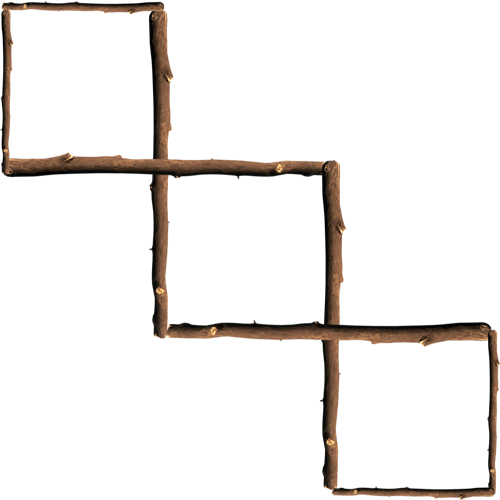A riddle problem is a question or statement intentionally phrased so as to require ingenuity in ascertaining its answer or meaning, typically presented as a game.  Below is a list of the past problem of the week riddles in descending chronological order.

Riddle: A horse is tied to a five-meter rope in front of an old saloon. Six meters behind the horse is a bale of hay. Without breaking his rope, the horse is able to eat the hay whenever he chooses. How is this possible?
Answer: The rope is not tied to anything else.
Riddle: I am a three digit number. My tens digit is five more than my ones digit. My hundreds digit is eight less than my tens digit. What number am I?
Riddle: Take eight small sticks, four of which are half the length of the other four. How can you make three equal squares out of the sticks?
Answer: Use the longer four sticks to be sharing sides between the squares and at the end their should be three intertwined squares.Riddle: There are 2 ducks in front of 2 other ducks. There are 2 ducks behind 2 other ducks. There are 2 ducks beside 2 other ducks. How many ducks are there?
Answer: Just four, in a square formation.
Riddle: Jim was examining an angle measuring 14 and 1/2 degrees, using his magnifying glass that magnifies everything two times. Under the glass, how large would that angle measure?
Answer: 14 and 1/2 degrees. Explanation, angles remain constant when magnified. A square has 4-90 degree corners, if you zoom in (magnify) a square, it's still a square.
Riddle: The ages of a father and son add up to 66. The father's age is the son's age reversed. How old could they be? (3 possible solutions).
Answer: 51 and 15. 42 and 24. 60 and 06.
Riddle: What arithmetic symbol can we place between 2 and 3 to make a number greater than 2 but less than 3?